Condition  (Pattn/;Test)

Condition is closely related to PatternTest.

Typical use of Condition

Condition is used to put a restriction on when a pattern is used and the syntax is forgiving.  Below I give three ways to use Condition to define a function that takes a positive number and return the square of the number.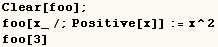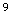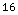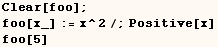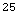In the next cell I show ho the same function can be defined using PatternTest.  It doesn't make much difference which style is used.  If you want to optimize running time, some experimenting is recommended.The next cell defines a function that takes a list of numbers and returns a list where each number is squared.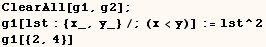The next cell shows an unsuccessful attempt to define the function that was defined in the previous input.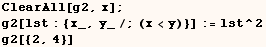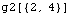The definition above failed because the global value of (x) was used in (y_/;(x<y)) instead of the value associated with (x_).  The definition that worked used the condition ({x_,y_}/;(x<y)) in which case all pattern variables used were in the pattern we had a condition on.  Notice the "broken" definition from the previous input is used when (x) has a suitable value.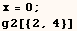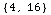A Subtle Point

David Park noted that using Condition in the definition of a function can give surprising results as demonstrated below.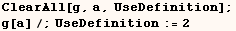In the next cell the definition of g[a] isn't used and this is what we expect.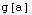Then in the next cell the definition of g[a] is used as we expect.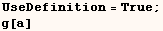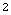Earlier the expression g[a] was assigned to expr, and one might expect that the following would evaluate to 2 since g[a]=2, but that isn't the case.Allan Hayes indicated this seems to stem from internal optimization procedures and he reminds us that section 2.5.12  of The Mathematica Book says the following. Some of the trickiest cases occur when you have rules that depend on complicated /; conditions (see Section 2.3.5). One particularly awkward case is when the condition involves a global variable. Mathematica may think that the evaluation is finished because the expression did not change. However, a side effect of some other operation could change the value of the global variable, and so should lead to a new result in the evaluation. The best way to avoid this kind of difficulty is not to use global variables in /; conditions. If all else fails, you can type Update[s] to tell Mathematica to update all expressions involving s.

Below Allan Hayes shows how we can define  expr  to ensure we get the expected result.
Here  Update  and  (lhs := rhs)  are used instead of  (lhs = rhs).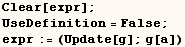In the last cell g[a] is returned when we had (UseDefinition = False).  In the next cell  we get  (expr→g[a]→2)  when (UseDefinition = True).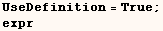Allan Hayes also gave the next solution which is slightly different from the one above, but gives the same result.Created by Mathematica  (May 16, 2004)

Back to Ted’s Tricks index page